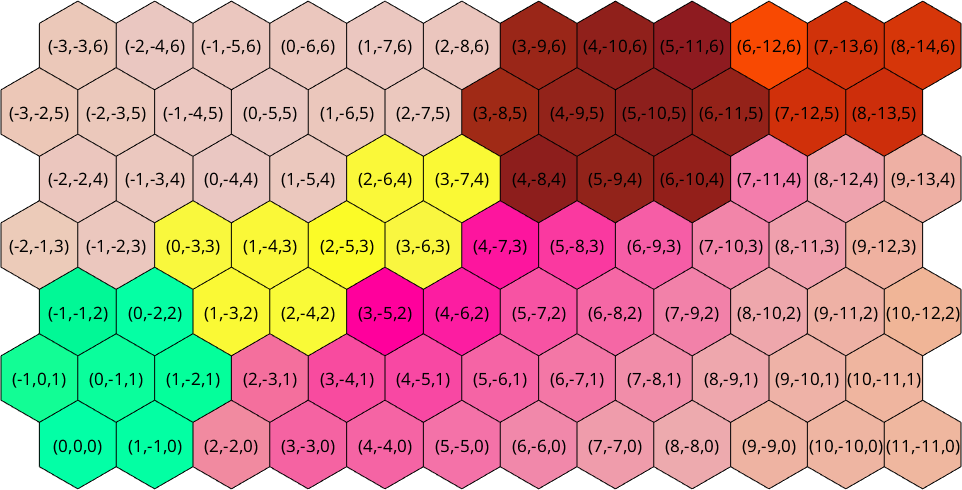The Index of EBSD data edit page

In previous chapters we have discussed how to select EBSD data by properties. In this chapter we are intested in the order the EBSD are stored within MTEX. Lets start by importing some sample data

and restricting it to very small rectangular subset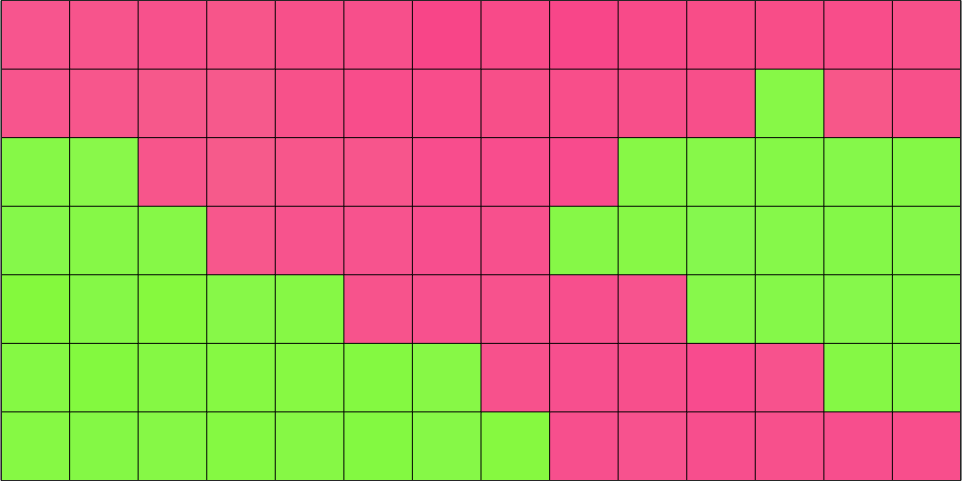In the above plot each square corresponds to one entry in the variable ebsd. Lets visualize the order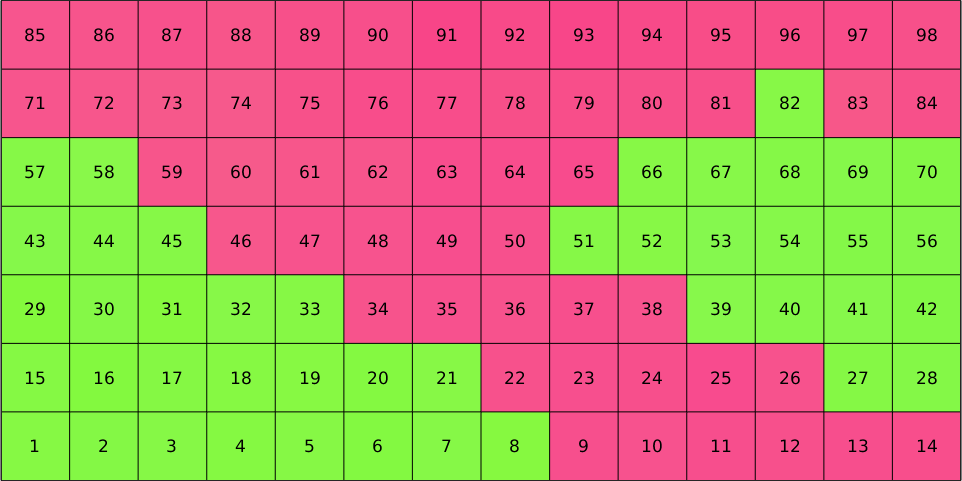We may easily select specific measurement pixels by specifying their indeces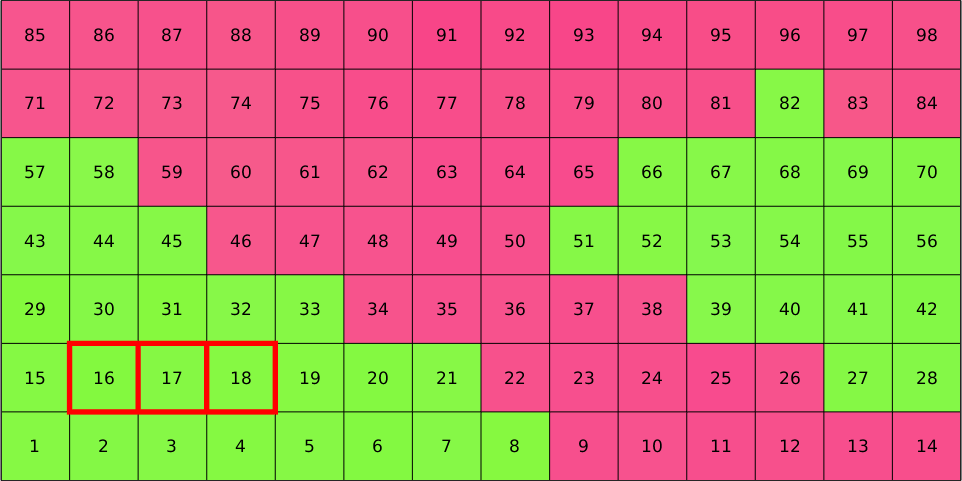Whether lines or columns run first is not related to MTEX but inherits from the ordering of the imported EBSD data. Since, we have restricted our large EBSD map to the small subset the indece of restricted data does not coincide with the indece of the imported data anymore. However, the original indeces are still stored in ebsd.id. Lets visualize them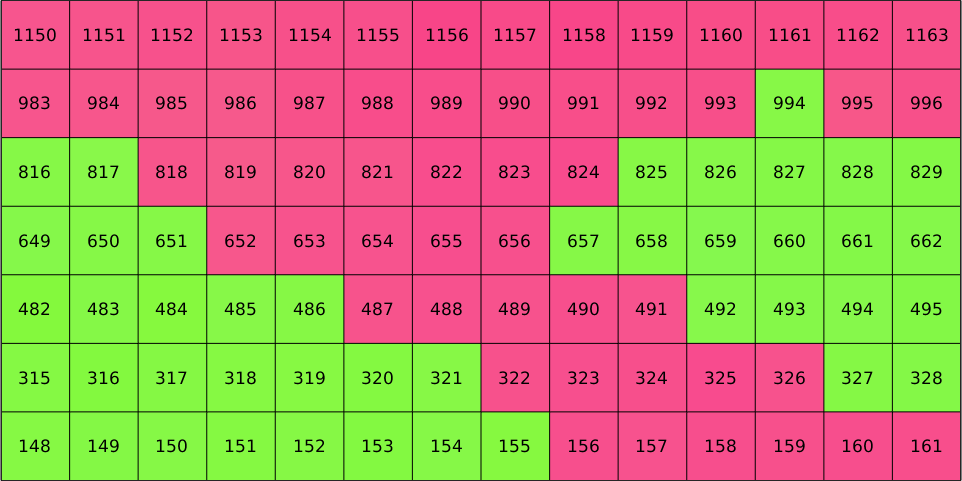In order to select EBSD data according to their original id use the option 'id', i.e.,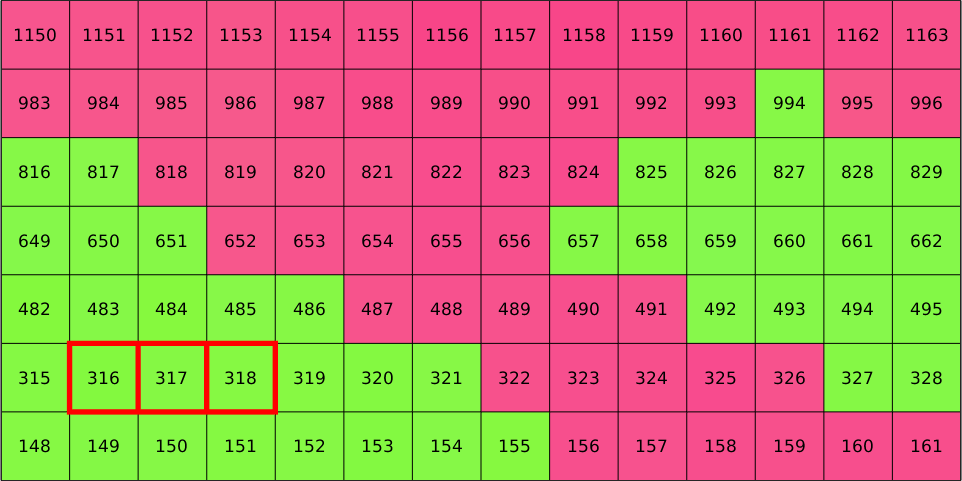## Square Grids

In the cases of gridded data it is often useful to convert them into a matrix form.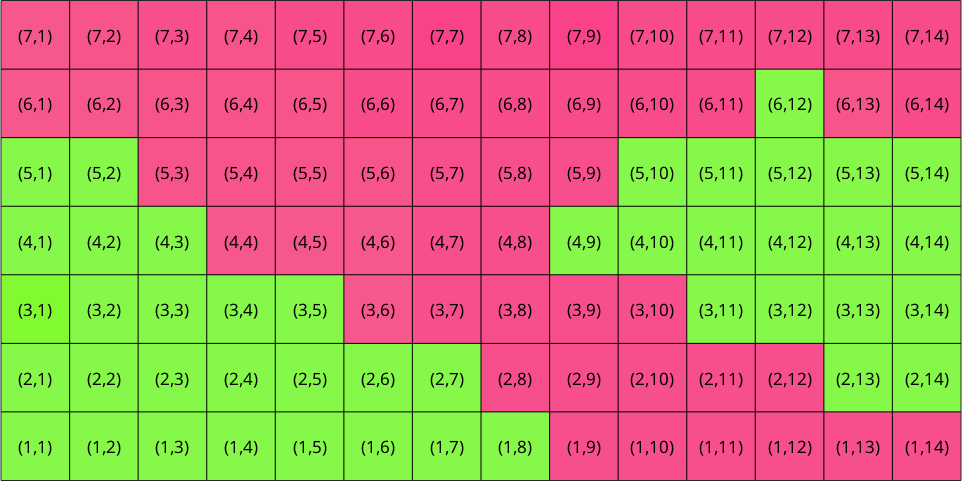This allows to select EBSD data simply by their coordinates within the grid, e.g., by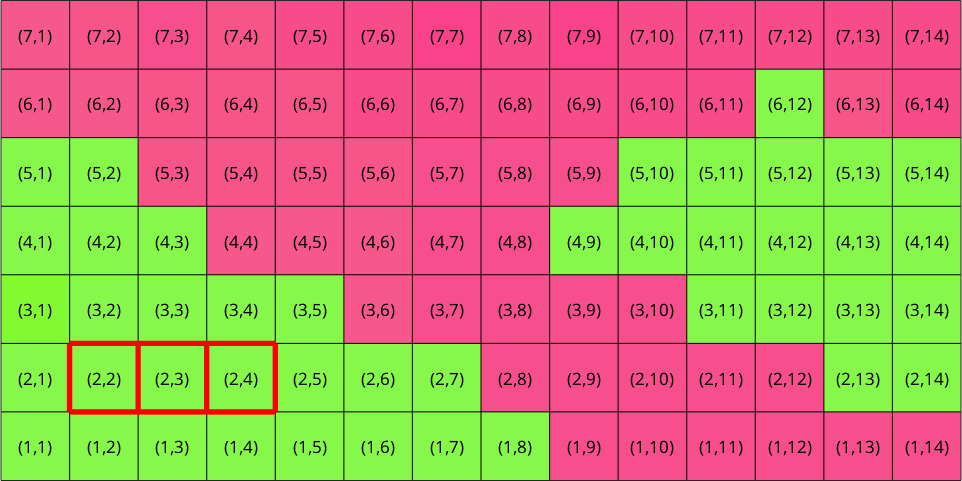Note that the gridify command changes the order of measurements. They are now sort such that rows runs first and columns second, as this is the default convention how Matlab indexes matrices.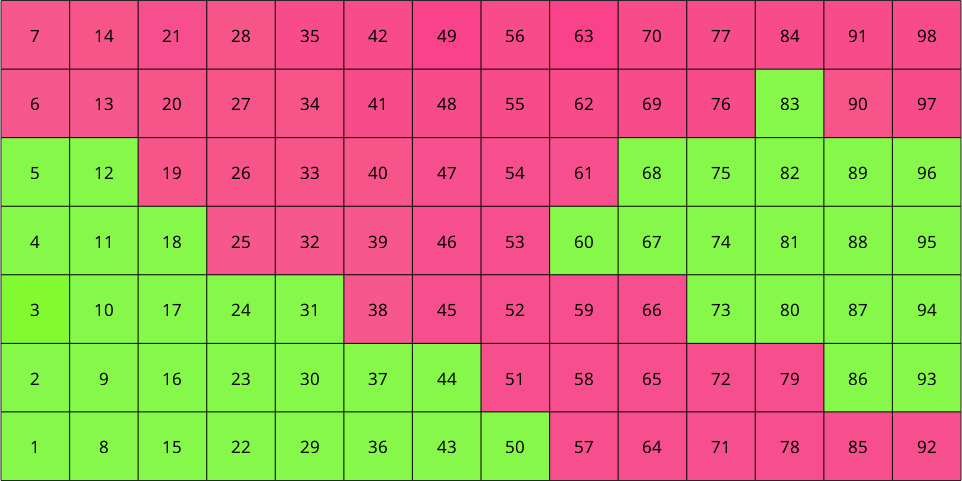## Hexagonal Grid

The command gridify may also be applied to EBSD data measured on a hexagonal grid.

This rearranges the measurements in a matrix form which can be indexed similarly as in the square case.

Lets visualize the matrix coordinates for the hexagonal grid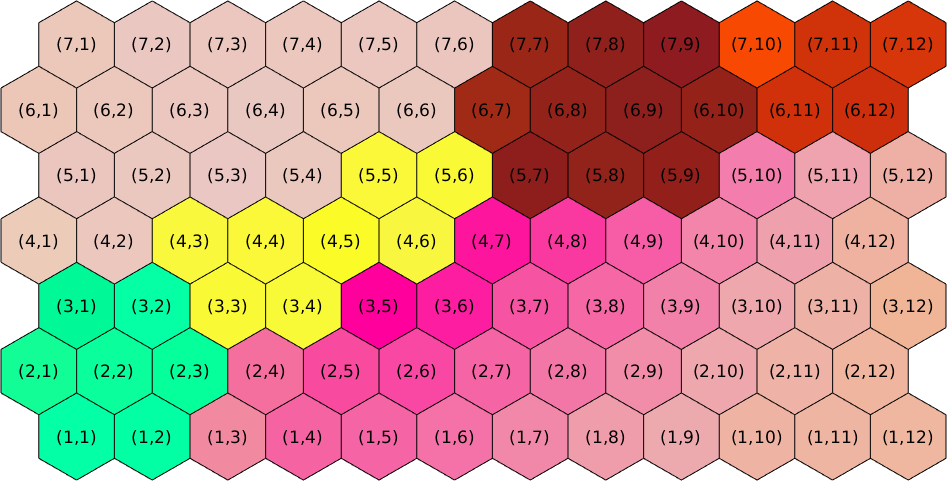## Cube Coordinates

In hexognal grids it is sometimes advantageous to use three digit cube coordinates to index the cell. This can be done using the commands hex2cube and cube2hex. Much more details on indexing hex grids can be found at here.